Скачать презентацию Session 2 Fundamentals and Classical methods of quantitative

c58523136e0eed5b82e37a4ef82c9441.ppt

• Количество слайдов: 45Session 2: Fundamentals and Classical methods of quantitative elemental analysis http: //bcs. whfreeman. com/qca 7 e http: //www. good-weighing-practice. com/gwp/proper-weighing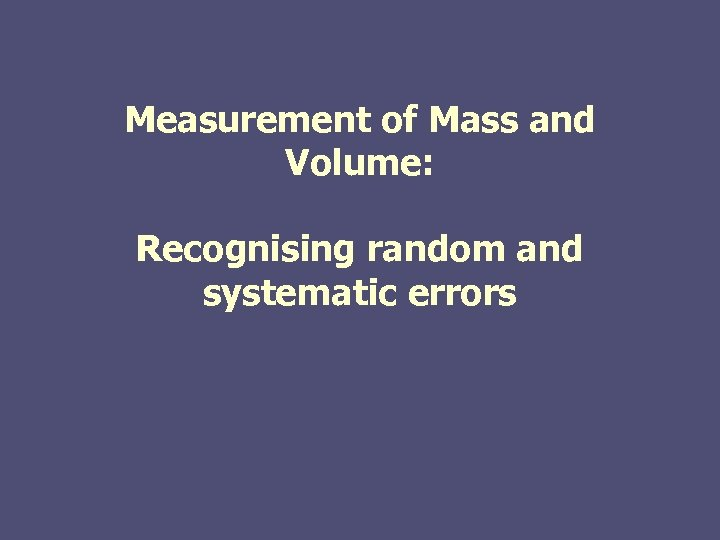Measurement of Mass and Volume: Recognising random and systematic errors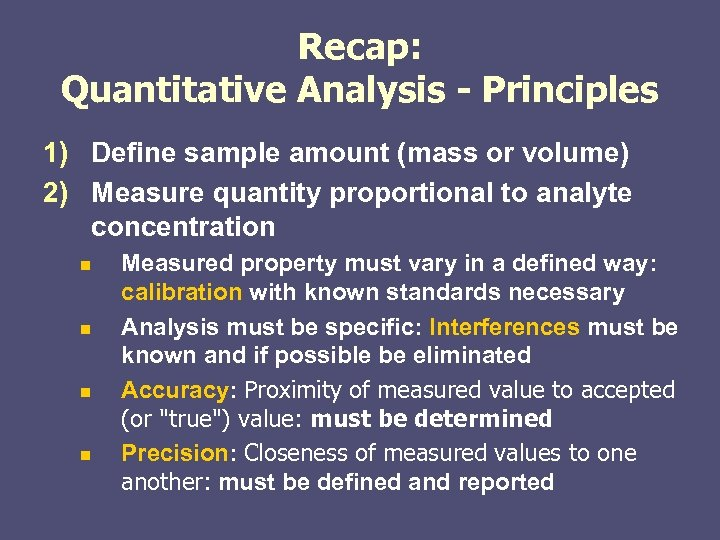Recap: Quantitative Analysis - Principles 1) Define sample amount (mass or volume) 2) Measure quantity proportional to analyte concentration n n Measured property must vary in a defined way: calibration with known standards necessary Analysis must be specific: Interferences must be known and if possible be eliminated Accuracy: Proximity of measured value to accepted (or "true") value: must be determined Precision: Closeness of measured values to one another: must be defined and reported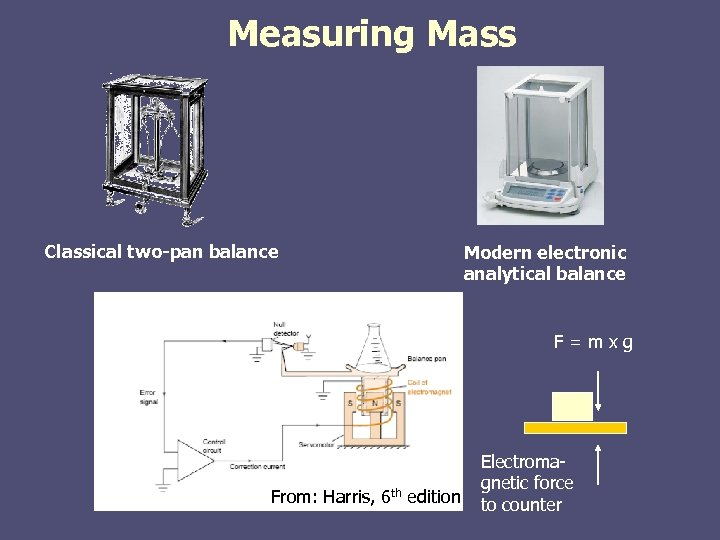Measuring Mass Classical two-pan balance Modern electronic analytical balance F=mxg From: Harris, 6 th edition Electromagnetic force to counter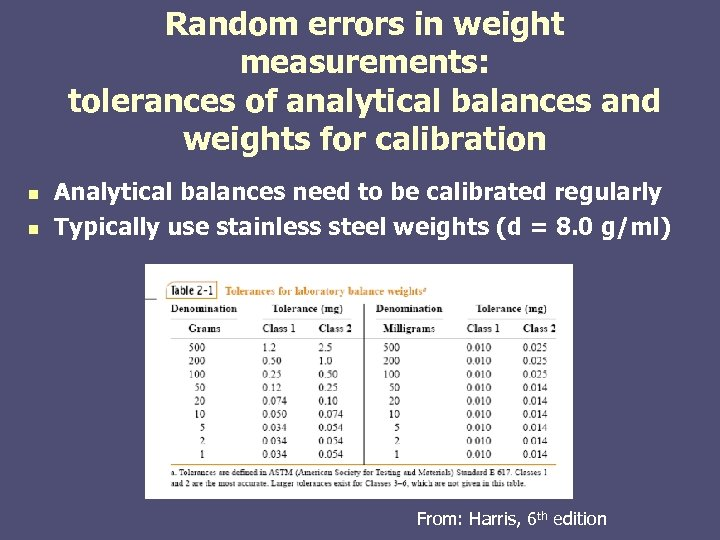Random errors in weight measurements: tolerances of analytical balances and weights for calibration n n Analytical balances need to be calibrated regularly Typically use stainless steel weights (d = 8. 0 g/ml) From: Harris, 6 th edition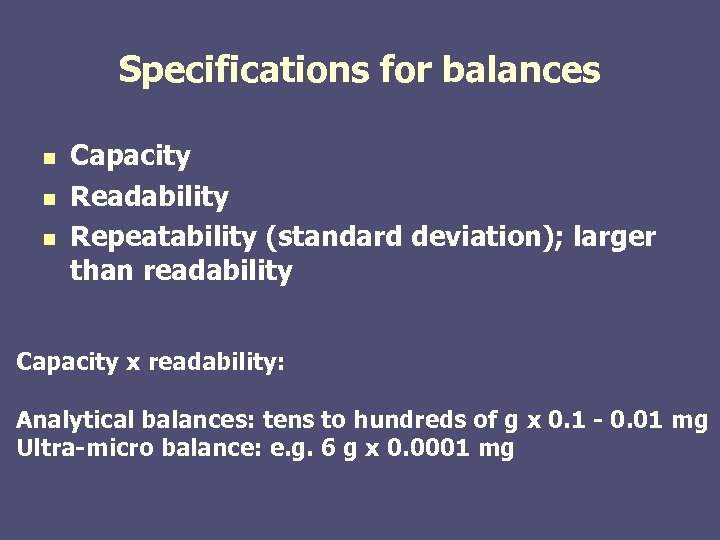Specifications for balances n n n Capacity Readability Repeatability (standard deviation); larger than readability Capacity x readability: Analytical balances: tens to hundreds of g x 0. 1 - 0. 01 mg Ultra-micro balance: e. g. 6 g x 0. 0001 mg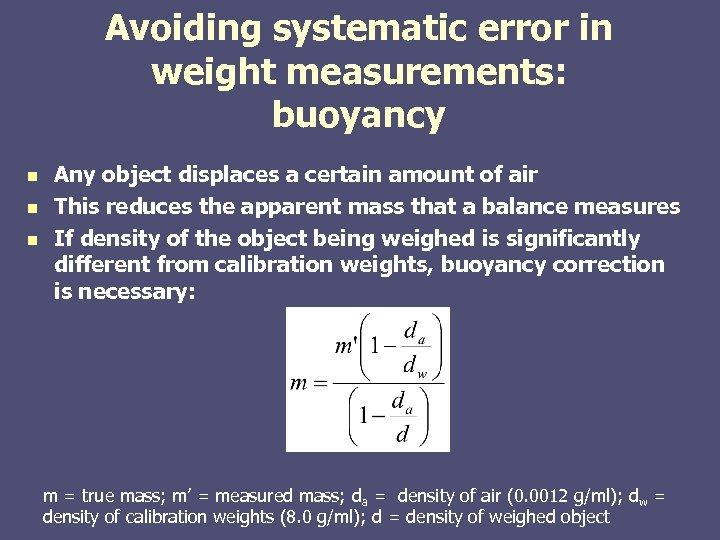Avoiding systematic error in weight measurements: buoyancy n n n Any object displaces a certain amount of air This reduces the apparent mass that a balance measures If density of the object being weighed is significantly different from calibration weights, buoyancy correction is necessary: m = true mass; m’ = measured mass; da = density of air (0. 0012 g/ml); dw = density of calibration weights (8. 0 g/ml); d = density of weighed object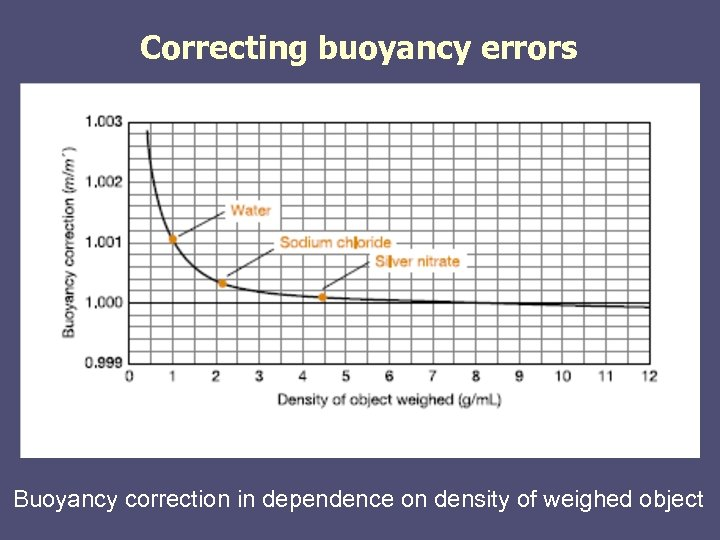Correcting buoyancy errors Buoyancy correction in dependence on density of weighed object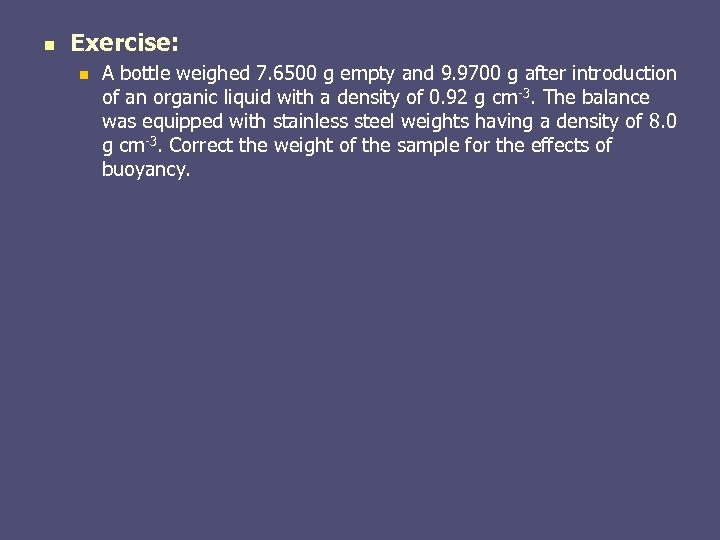n Exercise: n A bottle weighed 7. 6500 g empty and 9. 9700 g after introduction of an organic liquid with a density of 0. 92 g cm-3. The balance was equipped with stainless steel weights having a density of 8. 0 g cm-3. Correct the weight of the sample for the effects of buoyancy.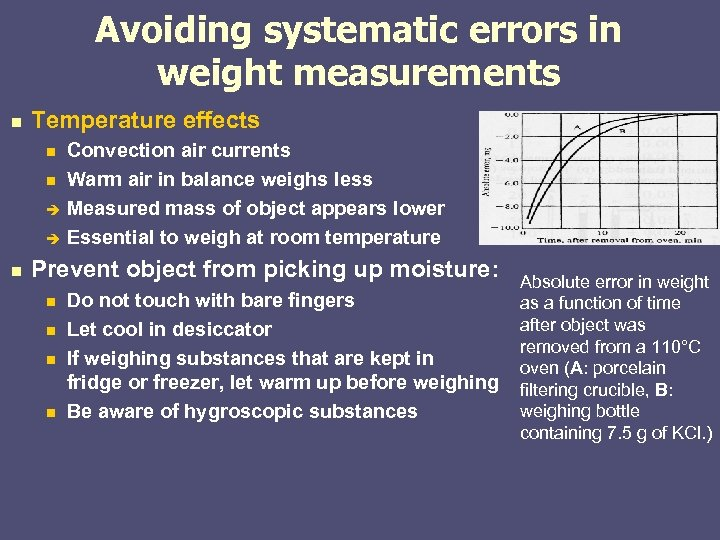Avoiding systematic errors in weight measurements n Temperature effects n n n Convection air currents Warm air in balance weighs less Measured mass of object appears lower Essential to weigh at room temperature Prevent object from picking up moisture: n n Do not touch with bare fingers Let cool in desiccator If weighing substances that are kept in fridge or freezer, let warm up before weighing Be aware of hygroscopic substances Absolute error in weight as a function of time after object was removed from a 110°C oven (A: porcelain filtering crucible, B: weighing bottle containing 7. 5 g of KCl. )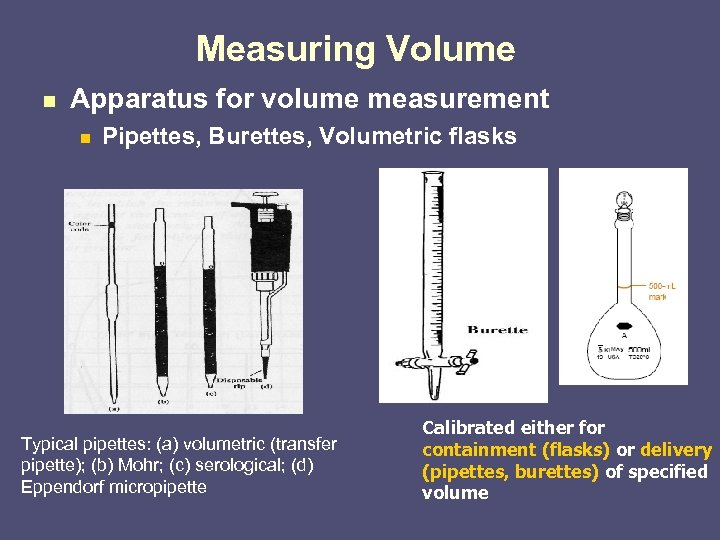Measuring Volume n Apparatus for volume measurement n Pipettes, Burettes, Volumetric flasks Typical pipettes: (a) volumetric (transfer pipette); (b) Mohr; (c) serological; (d) Eppendorf micropipette Calibrated either for containment (flasks) or delivery (pipettes, burettes) of specified volume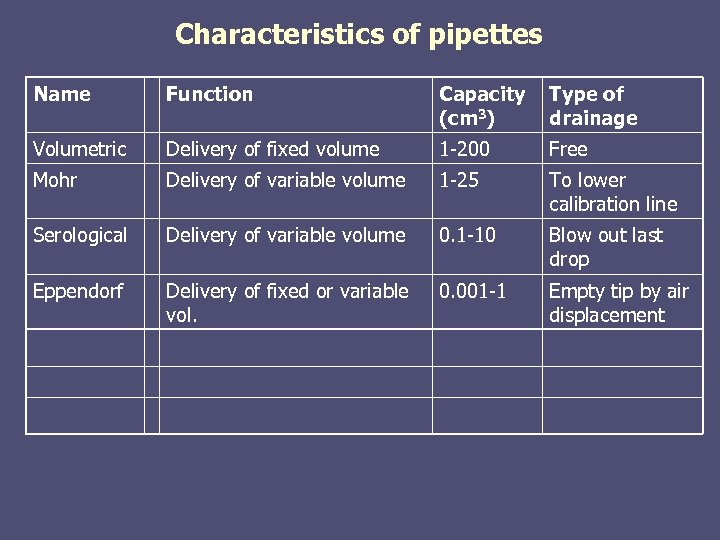Characteristics of pipettes Name Function Capacity (cm 3) Type of drainage Volumetric Delivery of fixed volume 1 -200 Free Mohr Delivery of variable volume 1 -25 To lower calibration line Serological Delivery of variable volume 0. 1 -10 Blow out last drop Eppendorf Delivery of fixed or variable vol. 0. 001 -1 Empty tip by air displacement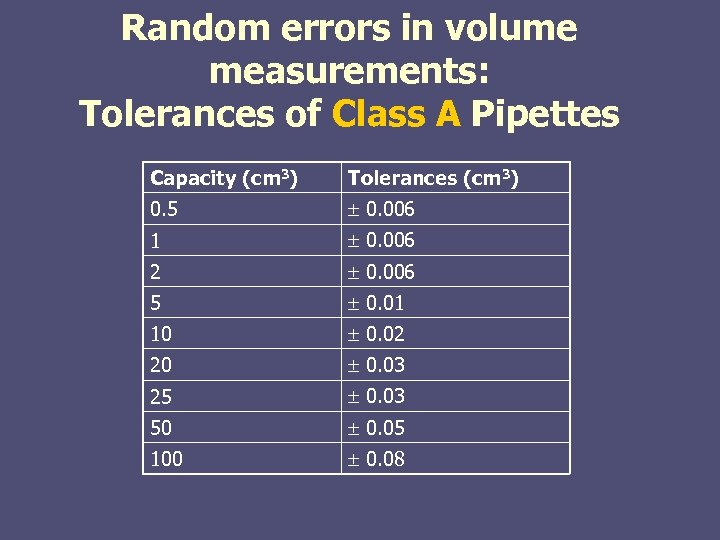Random errors in volume measurements: Tolerances of Class A Pipettes Capacity (cm 3) Tolerances (cm 3) 0. 5 0. 006 1 0. 006 2 0. 006 5 0. 01 10 0. 02 20 0. 03 25 0. 03 50 0. 05 100 0. 08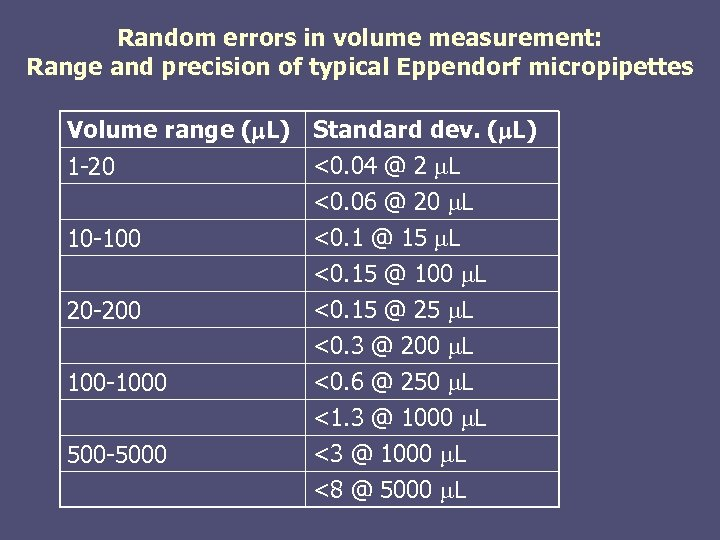Random errors in volume measurement: Range and precision of typical Eppendorf micropipettes Volume range (m. L) Standard dev. (m. L) <0. 04 @ 2 m. L 1 -20 <0. 06 @ 20 m. L <0. 1 @ 15 m. L 10 -100 20 -200 100 -1000 500 -5000 <0. 15 @ 100 m. L <0. 15 @ 25 m. L <0. 3 @ 200 m. L <0. 6 @ 250 m. L <1. 3 @ 1000 m. L <8 @ 5000 m. L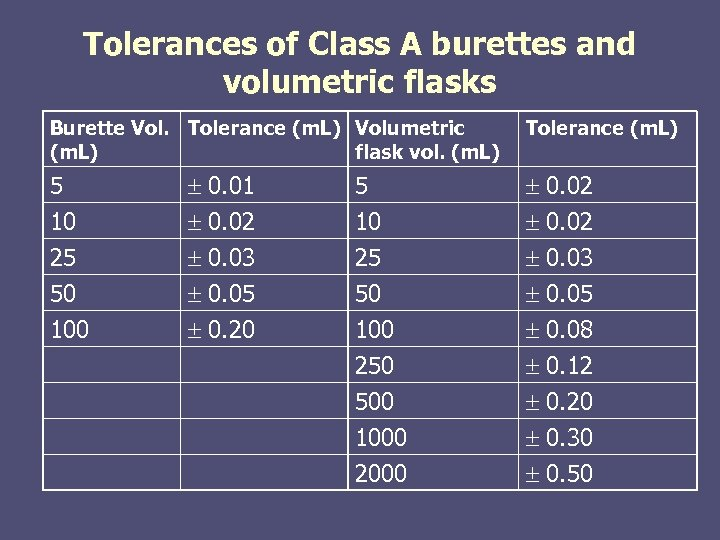Tolerances of Class A burettes and volumetric flasks Burette Vol. Tolerance (m. L) Volumetric (m. L) flask vol. (m. L) 5 10 25 50 100 0. 01 0. 02 0. 03 0. 05 0. 20 5 10 25 50 100 250 500 1000 2000 Tolerance (m. L) 0. 02 0. 03 0. 05 0. 08 0. 12 0. 20 0. 30 0. 50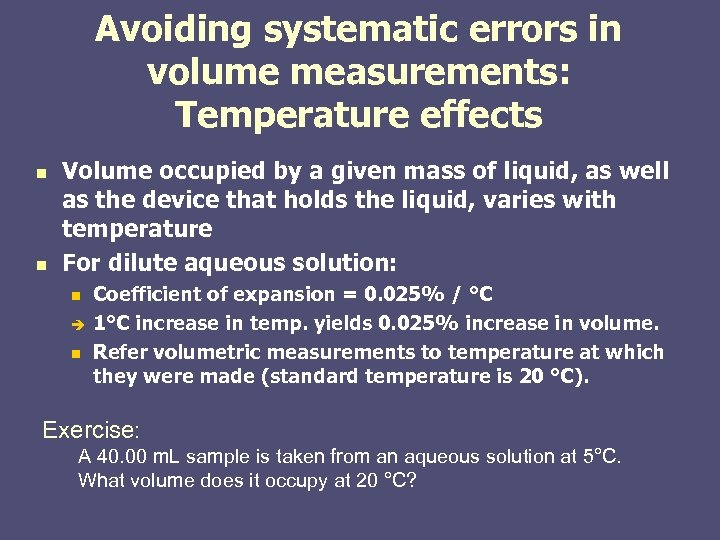Avoiding systematic errors in volume measurements: Temperature effects n n Volume occupied by a given mass of liquid, as well as the device that holds the liquid, varies with temperature For dilute aqueous solution: n n Coefficient of expansion = 0. 025% / °C 1°C increase in temp. yields 0. 025% increase in volume. Refer volumetric measurements to temperature at which they were made (standard temperature is 20 °C). Exercise: A 40. 00 m. L sample is taken from an aqueous solution at 5°C. What volume does it occupy at 20 °C?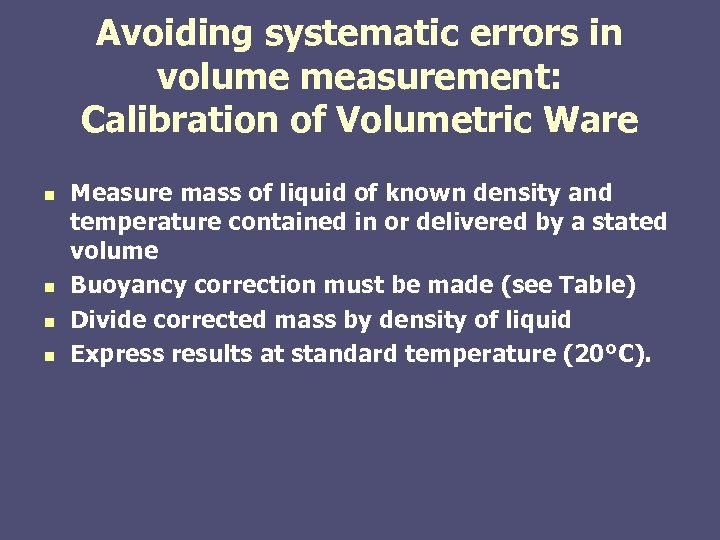Avoiding systematic errors in volume measurement: Calibration of Volumetric Ware n n Measure mass of liquid of known density and temperature contained in or delivered by a stated volume Buoyancy correction must be made (see Table) Divide corrected mass by density of liquid Express results at standard temperature (20°C).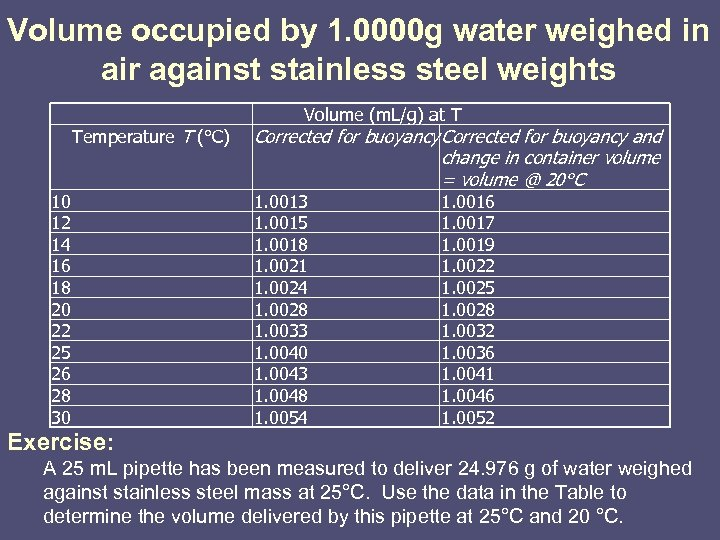Volume occupied by 1. 0000 g water weighed in air against stainless steel weights Temperature T (°C) 10 12 14 16 18 20 22 25 26 28 30 Volume (m. L/g) at T Corrected for buoyancy and change in container volume = volume @ 20°C 1. 0013 1. 0015 1. 0018 1. 0021 1. 0024 1. 0028 1. 0033 1. 0040 1. 0043 1. 0048 1. 0054 1. 0016 1. 0017 1. 0019 1. 0022 1. 0025 1. 0028 1. 0032 1. 0036 1. 0041 1. 0046 1. 0052 Exercise: A 25 m. L pipette has been measured to deliver 24. 976 g of water weighed against stainless steel mass at 25°C. Use the data in the Table to determine the volume delivered by this pipette at 25°C and 20 °C.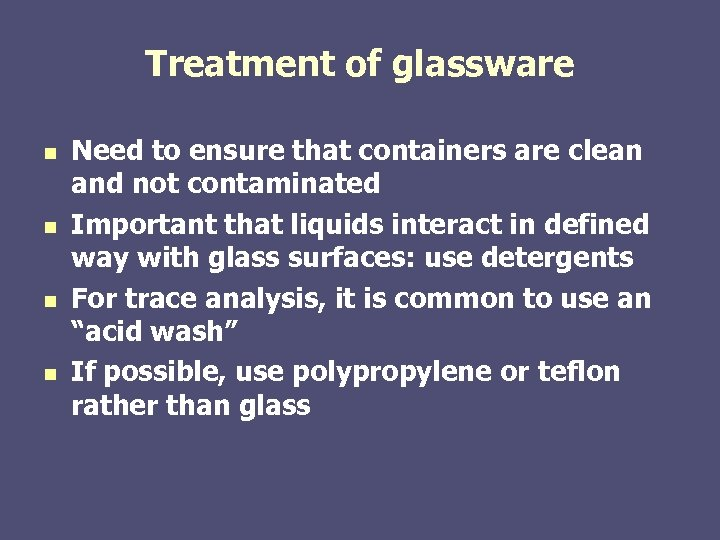Treatment of glassware n n Need to ensure that containers are clean and not contaminated Important that liquids interact in defined way with glass surfaces: use detergents For trace analysis, it is common to use an “acid wash” If possible, use polypropylene or teflon rather than glass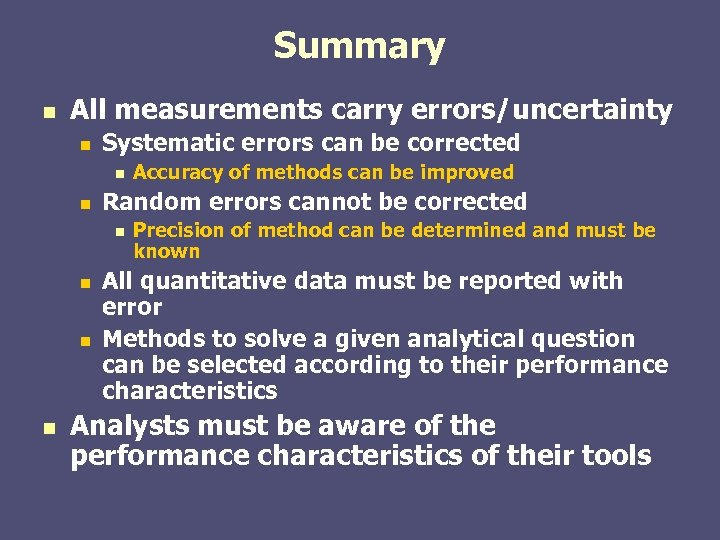Summary n All measurements carry errors/uncertainty n Systematic errors can be corrected n n Random errors cannot be corrected n n Accuracy of methods can be improved Precision of method can be determined and must be known All quantitative data must be reported with error Methods to solve a given analytical question can be selected according to their performance characteristics Analysts must be aware of the performance characteristics of their tools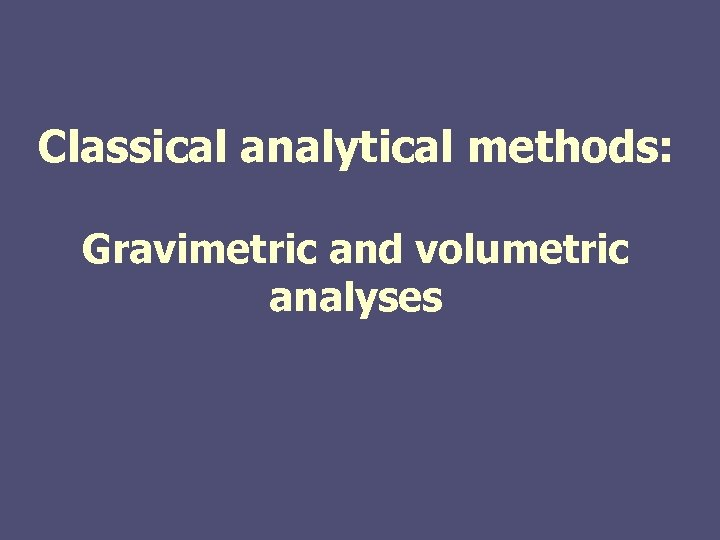Classical analytical methods: Gravimetric and volumetric analyses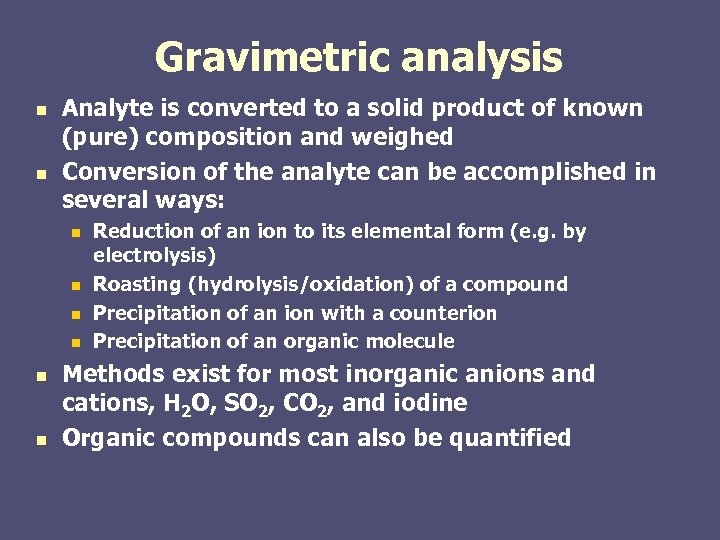Gravimetric analysis n n Analyte is converted to a solid product of known (pure) composition and weighed Conversion of the analyte can be accomplished in several ways: n n n Reduction of an ion to its elemental form (e. g. by electrolysis) Roasting (hydrolysis/oxidation) of a compound Precipitation of an ion with a counterion Precipitation of an organic molecule Methods exist for most inorganic anions and cations, H 2 O, SO 2, CO 2, and iodine Organic compounds can also be quantified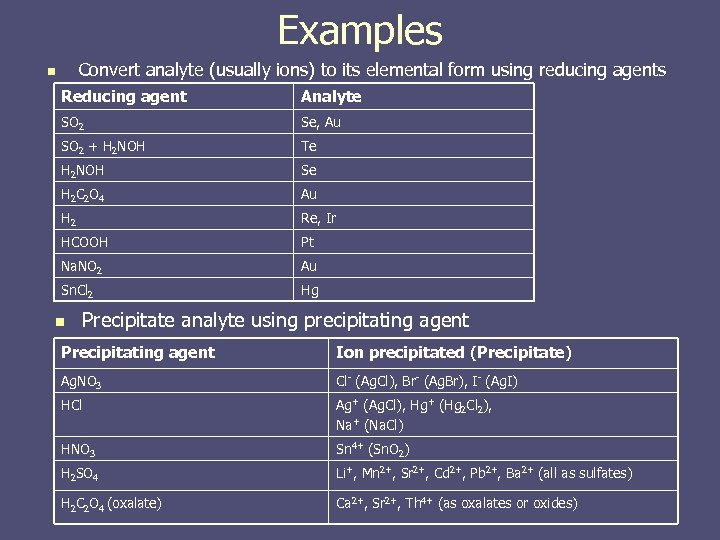Examples Convert analyte (usually ions) to its elemental form using reducing agents n Reducing agent Analyte SO 2 Se, Au SO 2 + H 2 NOH Te H 2 NOH Se H 2 C 2 O 4 Au H 2 Re, Ir HCOOH Pt Na. NO 2 Au Sn. Cl 2 Hg n Precipitate analyte using precipitating agent Precipitating agent Ion precipitated (Precipitate) Ag. NO 3 Cl- (Ag. Cl), Br- (Ag. Br), I- (Ag. I) HCl Ag+ (Ag. Cl), Hg+ (Hg 2 Cl 2), Na+ (Na. Cl) HNO 3 Sn 4+ (Sn. O 2) H 2 SO 4 Li+, Mn 2+, Sr 2+, Cd 2+, Pb 2+, Ba 2+ (all as sulfates) H 2 C 2 O 4 (oxalate) Ca 2+, Sr 2+, Th 4+ (as oxalates or oxides)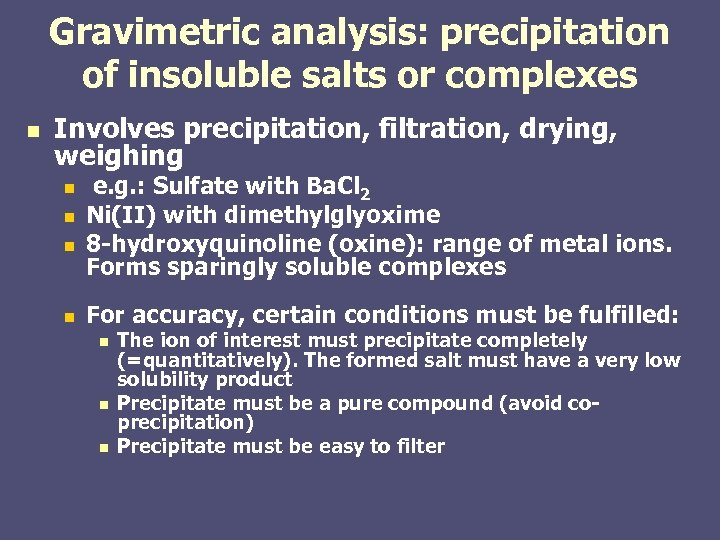Gravimetric analysis: precipitation of insoluble salts or complexes n Involves precipitation, filtration, drying, weighing n n e. g. : Sulfate with Ba. Cl 2 Ni(II) with dimethylglyoxime 8 -hydroxyquinoline (oxine): range of metal ions. Forms sparingly soluble complexes For accuracy, certain conditions must be fulfilled: n n n The ion of interest must precipitate completely (=quantitatively). The formed salt must have a very low solubility product Precipitate must be a pure compound (avoid coprecipitation) Precipitate must be easy to filter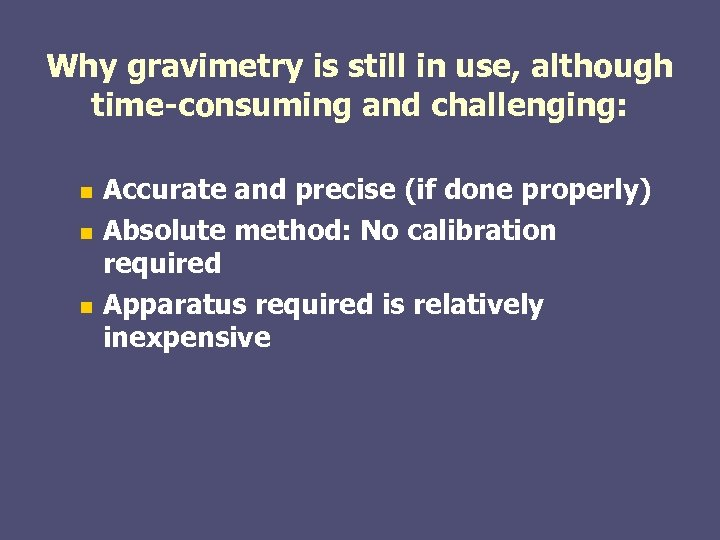Why gravimetry is still in use, although time-consuming and challenging: Accurate and precise (if done properly) n Absolute method: No calibration required n Apparatus required is relatively inexpensive n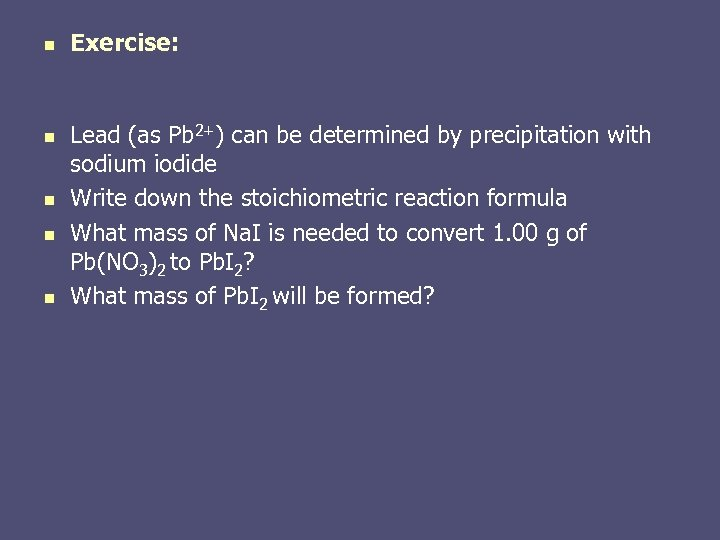n n n Exercise: Lead (as Pb 2+) can be determined by precipitation with sodium iodide Write down the stoichiometric reaction formula What mass of Na. I is needed to convert 1. 00 g of Pb(NO 3)2 to Pb. I 2? What mass of Pb. I 2 will be formed?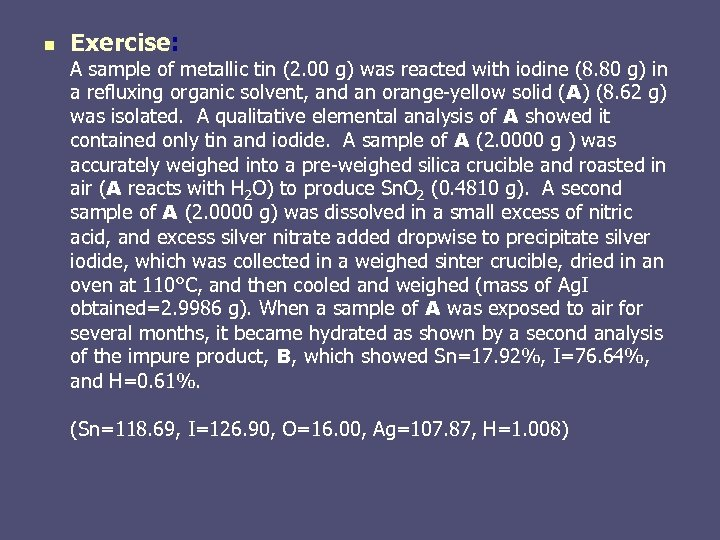n Exercise: A sample of metallic tin (2. 00 g) was reacted with iodine (8. 80 g) in a refluxing organic solvent, and an orange-yellow solid ( A) (8. 62 g) was isolated. A qualitative elemental analysis of A showed it contained only tin and iodide. A sample of A (2. 0000 g ) was accurately weighed into a pre-weighed silica crucible and roasted in air (A reacts with H 2 O) to produce Sn. O 2 (0. 4810 g). A second sample of A (2. 0000 g) was dissolved in a small excess of nitric acid, and excess silver nitrate added dropwise to precipitate silver iodide, which was collected in a weighed sinter crucible, dried in an oven at 110°C, and then cooled and weighed (mass of Ag. I obtained=2. 9986 g). When a sample of A was exposed to air for several months, it became hydrated as shown by a second analysis of the impure product, B, which showed Sn=17. 92%, I=76. 64%, and H=0. 61%. (Sn=118. 69, I=126. 90, O=16. 00, Ag=107. 87, H=1. 008)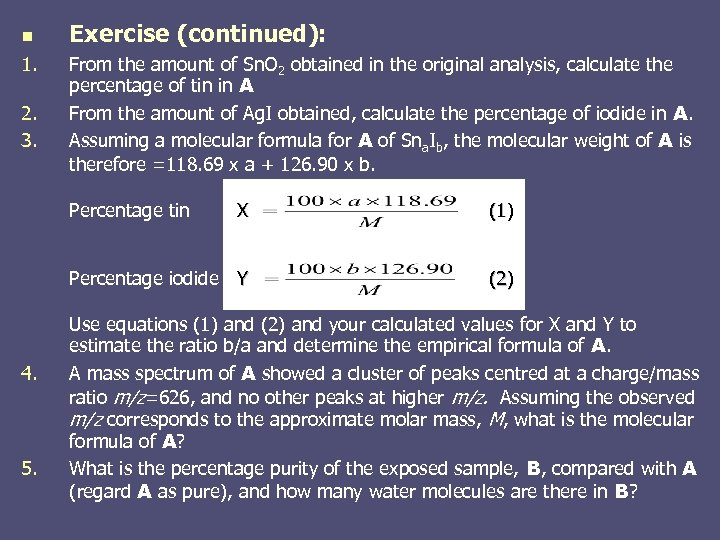n 1. 2. 3. Exercise (continued): From the amount of Sn. O 2 obtained in the original analysis, calculate the percentage of tin in A From the amount of Ag. I obtained, calculate the percentage of iodide in A. Assuming a molecular formula for A of Sna. Ib, the molecular weight of A is therefore =118. 69 x a + 126. 90 x b. Percentage tin 5. (1) Percentage iodide Y 4. X (2) Use equations (1) and (2) and your calculated values for X and Y to estimate the ratio b/a and determine the empirical formula of A. A mass spectrum of A showed a cluster of peaks centred at a charge/mass ratio m/z=626, and no other peaks at higher m/z. Assuming the observed m/z corresponds to the approximate molar mass, M, what is the molecular formula of A? What is the percentage purity of the exposed sample, B, compared with A (regard A as pure), and how many water molecules are there in B?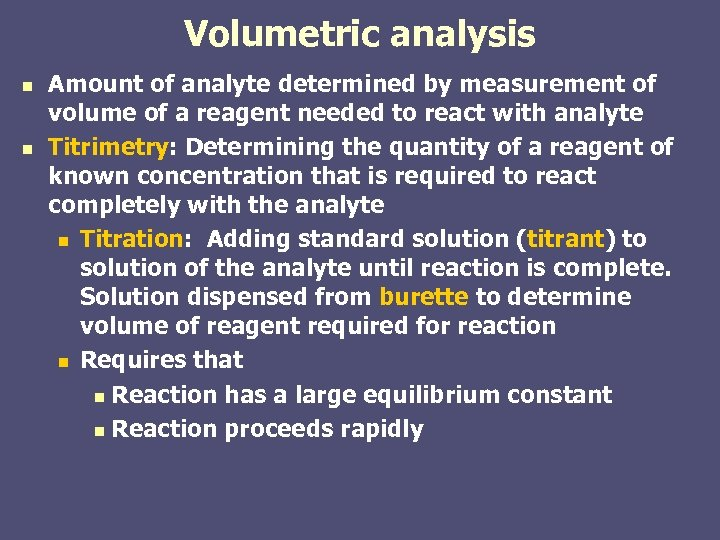Volumetric analysis n n Amount of analyte determined by measurement of volume of a reagent needed to react with analyte Titrimetry: Determining the quantity of a reagent of known concentration that is required to react completely with the analyte n Titration: Adding standard solution (titrant) to solution of the analyte until reaction is complete. Solution dispensed from burette to determine volume of reagent required for reaction n Requires that n Reaction has a large equilibrium constant n Reaction proceeds rapidly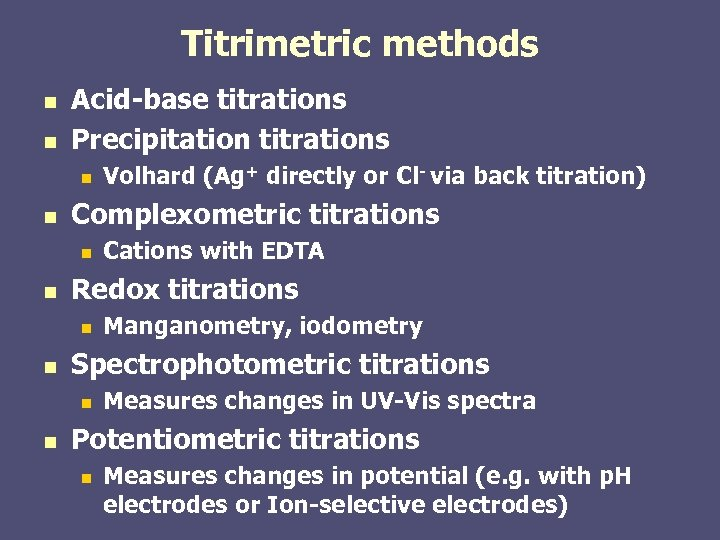Titrimetric methods n n Acid-base titrations Precipitation titrations n n Complexometric titrations n n Manganometry, iodometry Spectrophotometric titrations n n Cations with EDTA Redox titrations n n Volhard (Ag+ directly or Cl- via back titration) Measures changes in UV-Vis spectra Potentiometric titrations n Measures changes in potential (e. g. with p. H electrodes or Ion-selective electrodes)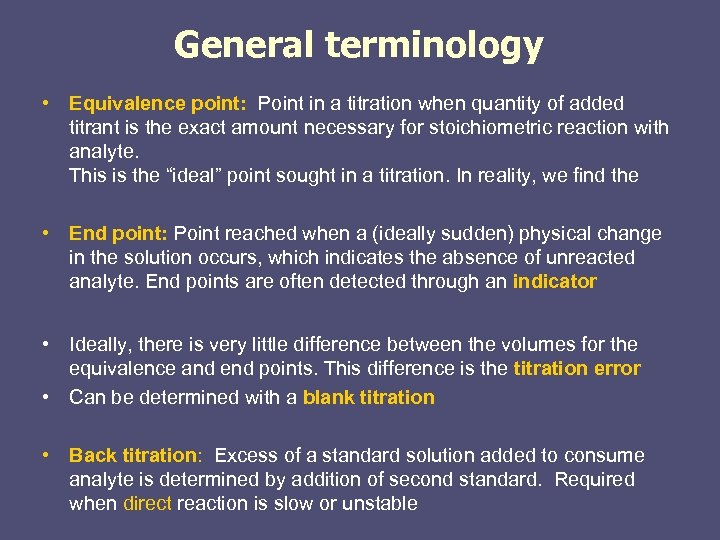General terminology • Equivalence point: Point in a titration when quantity of added titrant is the exact amount necessary for stoichiometric reaction with analyte. This is the “ideal” point sought in a titration. In reality, we find the • End point: Point reached when a (ideally sudden) physical change in the solution occurs, which indicates the absence of unreacted analyte. End points are often detected through an indicator • Ideally, there is very little difference between the volumes for the equivalence and end points. This difference is the titration error • Can be determined with a blank titration • Back titration: Excess of a standard solution added to consume analyte is determined by addition of second standard. Required when direct reaction is slow or unstable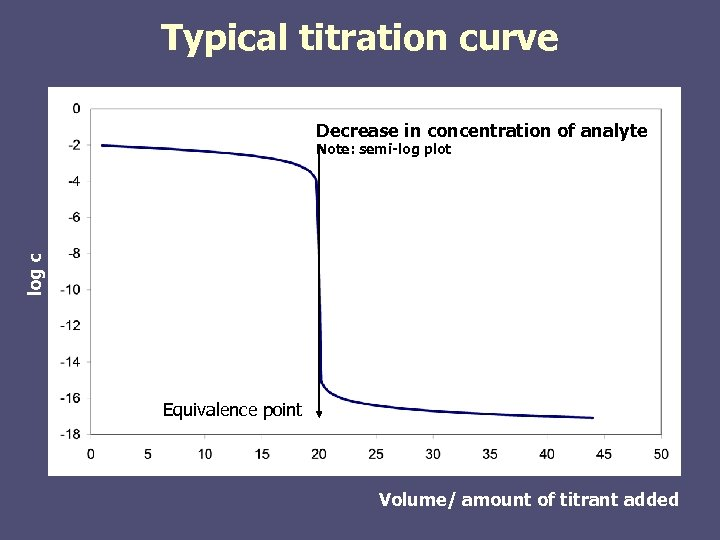Typical titration curve Decrease in concentration of analyte log c Note: semi-log plot Equivalence point Volume/ amount of titrant added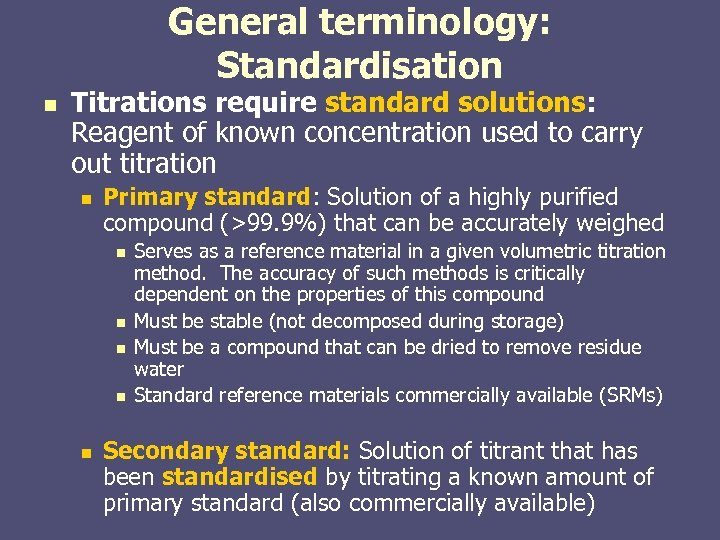General terminology: Standardisation n Titrations require standard solutions: Reagent of known concentration used to carry out titration n Primary standard: Solution of a highly purified compound (>99. 9%) that can be accurately weighed n n n Serves as a reference material in a given volumetric titration method. The accuracy of such methods is critically dependent on the properties of this compound Must be stable (not decomposed during storage) Must be a compound that can be dried to remove residue water Standard reference materials commercially available (SRMs) Secondary standard: Solution of titrant that has been standardised by titrating a known amount of primary standard (also commercially available)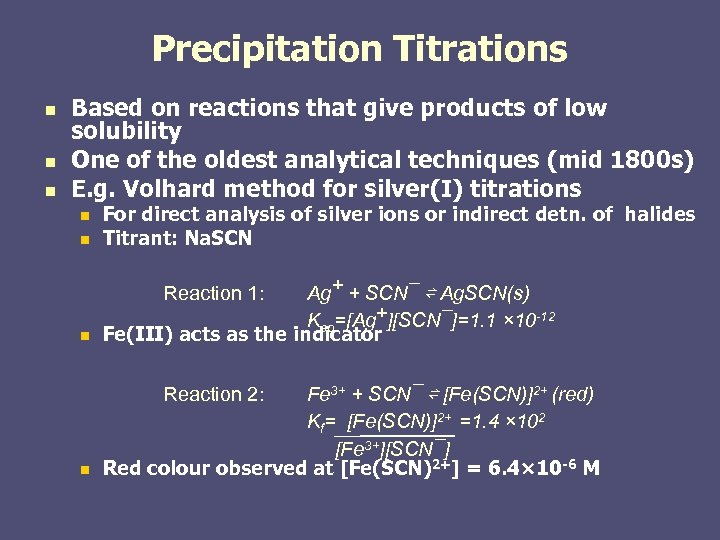Precipitation Titrations n n n Based on reactions that give products of low solubility One of the oldest analytical techniques (mid 1800 s) E. g. Volhard method for silver(I) titrations n For direct analysis of silver ions or indirect detn. of halides Titrant: Na. SCN n Ag+ + SCN¯ ⇌ Ag. SCN(s) Ksp=[Ag+][SCN¯]=1. 1 × 10 -12 Fe(III) acts as the indicator n Fe 3+ + SCN¯ ⇌ [Fe(SCN)]2+ (red) Kf= [Fe(SCN)]2+ =1. 4 × 102 [Fe 3+][SCN¯] Red colour observed at [Fe(SCN)2+] = 6. 4× 10 -6 M n Reaction 1: Reaction 2: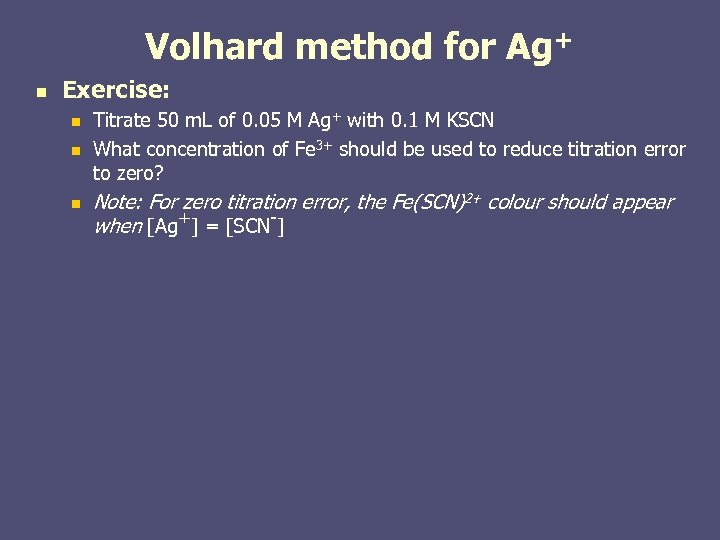Volhard method for Ag+ n Exercise: n n n Titrate 50 m. L of 0. 05 M Ag+ with 0. 1 M KSCN What concentration of Fe 3+ should be used to reduce titration error to zero? Note: For zero titration error, the Fe(SCN)2+ colour should appear when [Ag+] = [SCN-]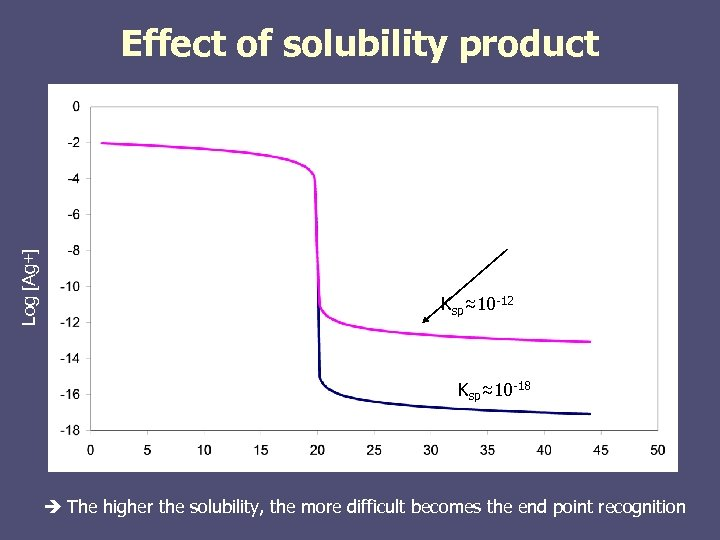Log [Ag+] Effect of solubility product Ksp≈10 -12 Ksp≈10 -18 The higher the solubility, the more difficult becomes the end point recognition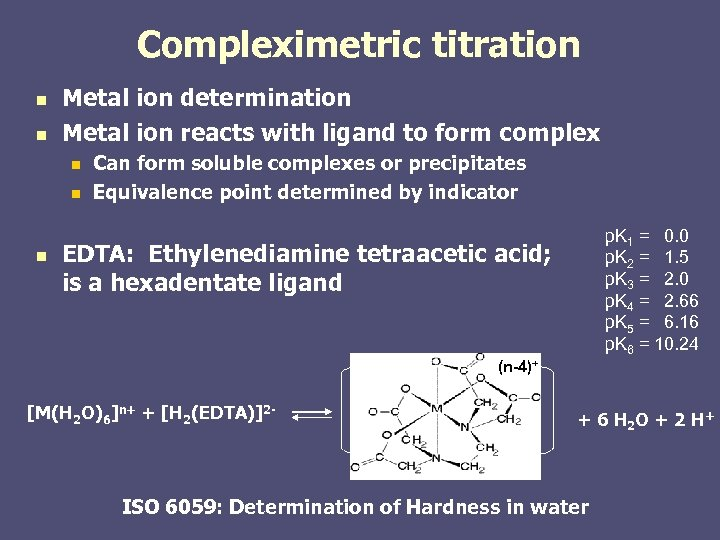Compleximetric titration n n Metal ion determination Metal ion reacts with ligand to form complex n n n Can form soluble complexes or precipitates Equivalence point determined by indicator p. K 1 = 0. 0 p. K 2 = 1. 5 p. K 3 = 2. 0 p. K 4 = 2. 66 p. K 5 = 6. 16 p. K 6 = 10. 24 EDTA: Ethylenediamine tetraacetic acid; is a hexadentate ligand (n-4)+ [M(H 2 O)6]n+ + [H 2(EDTA)]2 - + 6 H 2 O + 2 H + ISO 6059: Determination of Hardness in water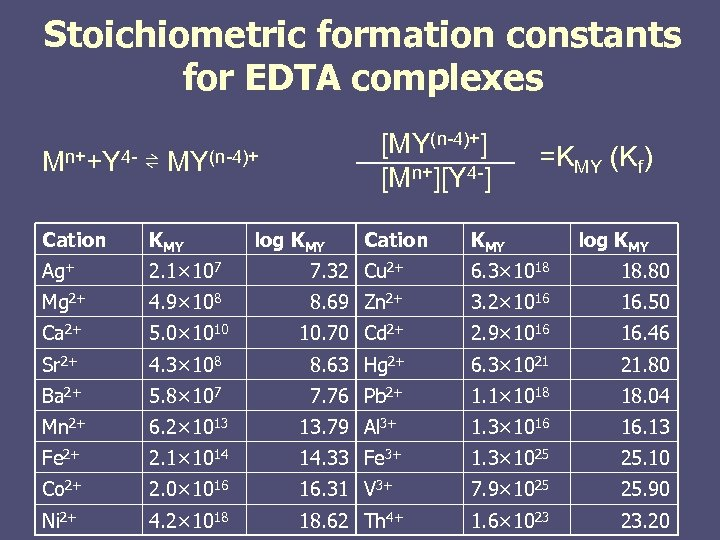Stoichiometric formation constants for EDTA complexes [MY(n-4)+] [Mn+][Y 4 -] Mn++Y 4 - ⇌ MY(n-4)+ log KMY Cation =KMY (Kf) Cation KMY log KMY Ag+ 2. 1× 107 7. 32 Cu 2+ 6. 3× 1018 18. 80 Mg 2+ 4. 9× 108 8. 69 Zn 2+ 3. 2× 1016 16. 50 Ca 2+ 5. 0× 1010 10. 70 Cd 2+ 2. 9× 1016 16. 46 Sr 2+ 4. 3× 108 8. 63 Hg 2+ 6. 3× 1021 21. 80 Ba 2+ 5. 8× 107 7. 76 Pb 2+ 1. 1× 1018 18. 04 Mn 2+ 6. 2× 1013 13. 79 Al 3+ 1. 3× 1016 16. 13 Fe 2+ 2. 1× 1014 14. 33 Fe 3+ 1. 3× 1025 25. 10 Co 2+ 2. 0× 1016 16. 31 V 3+ 7. 9× 1025 25. 90 Ni 2+ 4. 2× 1018 18. 62 Th 4+ 1. 6× 1023 23. 20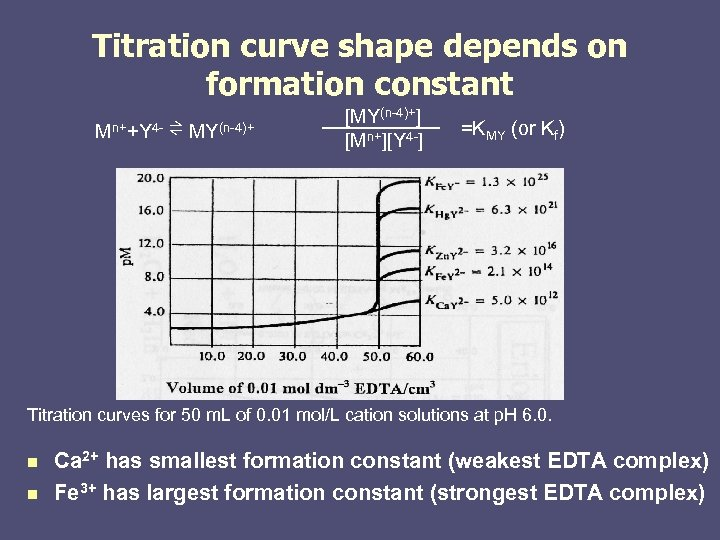Titration curve shape depends on formation constant Mn++Y 4 - ⇌ MY(n-4)+ [MY(n-4)+] [Mn+][Y 4 -] =KMY (or Kf) Titration curves for 50 m. L of 0. 01 mol/L cation solutions at p. H 6. 0. n n Ca 2+ has smallest formation constant (weakest EDTA complex) Fe 3+ has largest formation constant (strongest EDTA complex)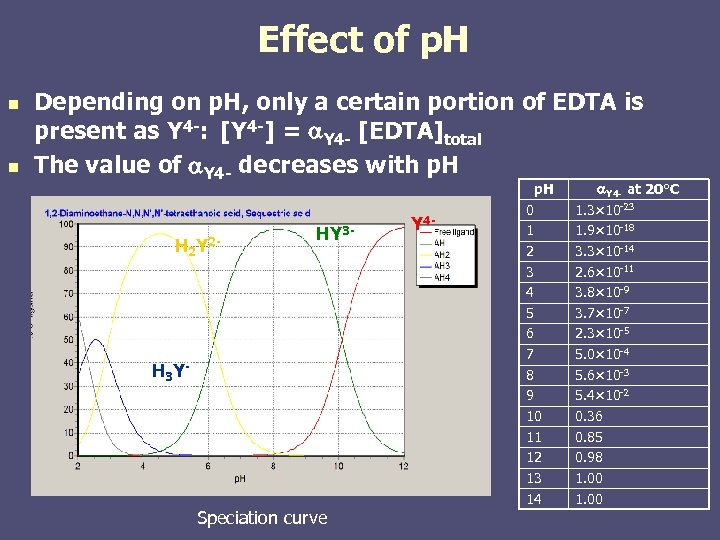Effect of p. H n n Depending on p. H, only a certain portion of EDTA is present as Y 4 -: [Y 4 -] = a. Y 4 - [EDTA]total The value of a. Y 4 - decreases with p. H H 2 Y 2 - HY 3 - H 3 Y - Speciation curve Y 4 - p. H 0 1 2 3 4 5 6 7 8 9 10 11 12 13 14 a. Y 4 - at 20°C 1. 3× 10 -23 1. 9× 10 -18 3. 3× 10 -14 2. 6× 10 -11 3. 8× 10 -9 3. 7× 10 -7 2. 3× 10 -5 5. 0× 10 -4 5. 6× 10 -3 5. 4× 10 -2 0. 36 0. 85 0. 98 1. 00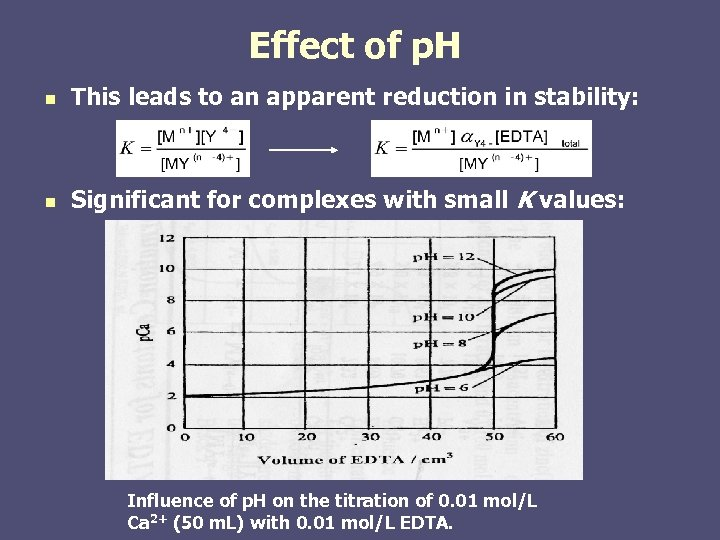Effect of p. H n This leads to an apparent reduction in stability: n Significant for complexes with small K values: Influence of p. H on the titration of 0. 01 mol/L Ca 2+ (50 m. L) with 0. 01 mol/L EDTA.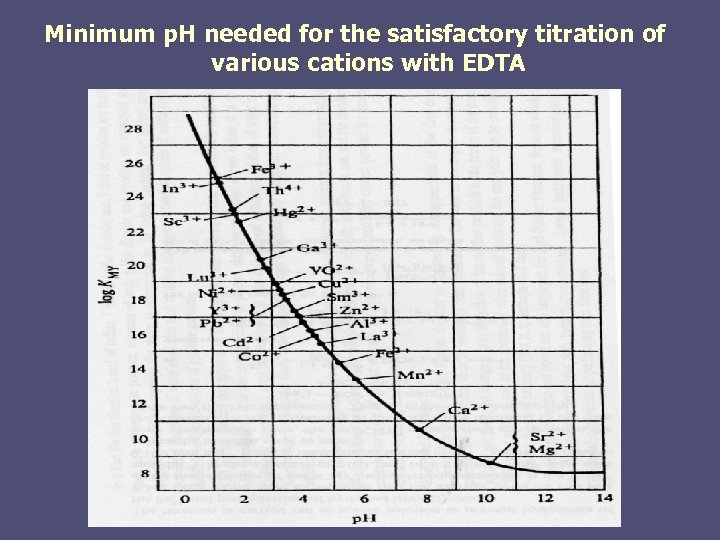Minimum p. H needed for the satisfactory titration of various cations with EDTA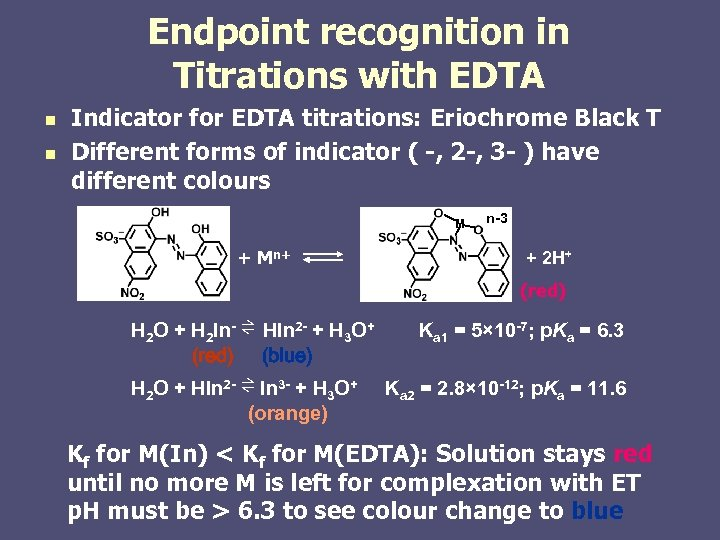Endpoint recognition in Titrations with EDTA n n Indicator for EDTA titrations: Eriochrome Black T Different forms of indicator ( -, 2 -, 3 - ) have different colours M + Mn+ n-3 + 2 H+ (red) H 2 O + H 2 In- ⇌ HIn 2 - + H 3 O+ (red) (blue) H 2 O + HIn 2 - ⇌ In 3 - + H 3 O+ (orange) Ka 1 = 5× 10 -7; p. Ka = 6. 3 Ka 2 = 2. 8× 10 -12; p. Ka = 11. 6 Kf for M(In) < Kf for M(EDTA): Solution stays red until no more M is left for complexation with ET p. H must be > 6. 3 to see colour change to blue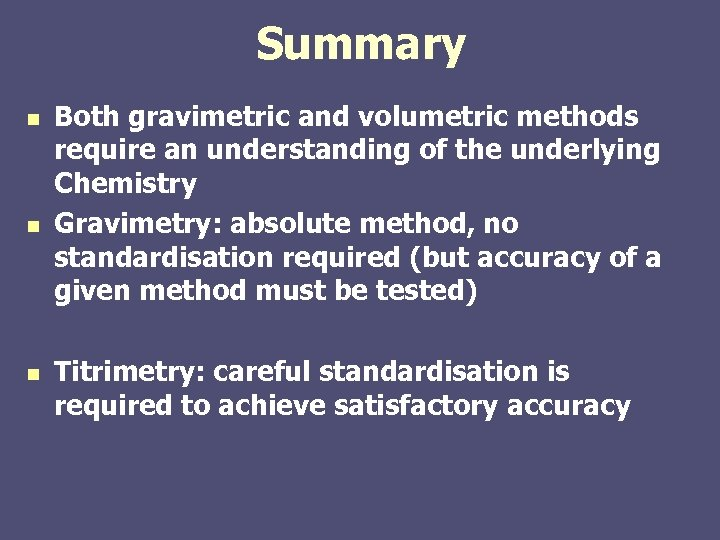Summary n n n Both gravimetric and volumetric methods require an understanding of the underlying Chemistry Gravimetry: absolute method, no standardisation required (but accuracy of a given method must be tested) Titrimetry: careful standardisation is required to achieve satisfactory accuracy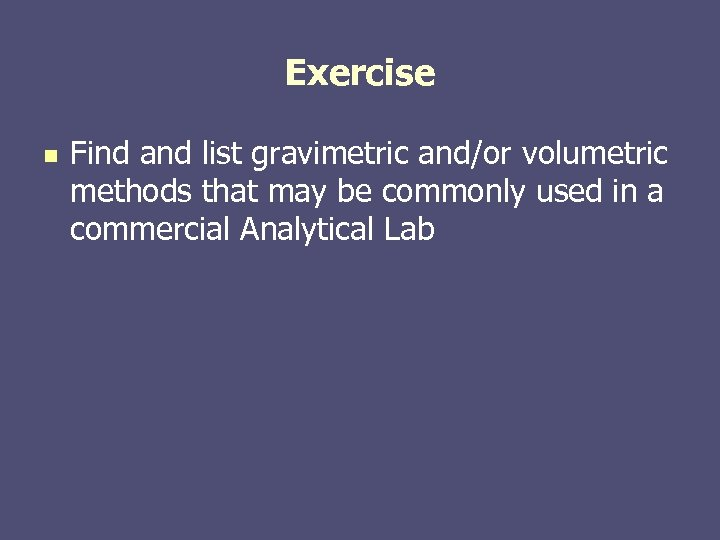Exercise n Find and list gravimetric and/or volumetric methods that may be commonly used in a commercial Analytical Lab